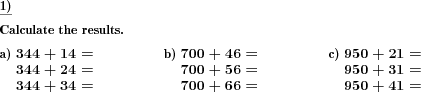Custom math worksheets at your fingertips# Details for problem "Add and subtract terms with incrementing summand"

Quickname: 5355

Elementary School, Primary School.

## Summary

In a series of addition or subtraction terms, one number changes stepwise.

## Example## Description

A series of additon or subtraction terms is given, arranged in an column. The first number (first summand or minuend) stays the same, while the second number (second summand or subtrahend) increases or decreases in a single digit position.

The second number is always one digit less in size.

The number of digits of the first (and by that, the second) number is selectable, and so is the number of terms in a series. The stepping direction may be chosen as up for an increasing second number or down for a decreasing one. It can be chosen whether the changing digit is always the first (highest order) one or any digit may be the changing one. In any case, the digit is fixed for each series of terms.

By selection only addition or only subtraction terms will be presented, or a mixture of both. As a simplification, it may be specified that there will be no borrows or carries over the second number's digits.

There is a setting that trap questions may be included, where occasionally, the first number of a term will change, to catch candidates who just write down a plain sequence on the right hand side without reading the task.

Download free printable worksheets for this math problem here. The worksheet contains the problems only, the solution sheet includes the answers. Just click on the respective link.

•Worksheet 1Solution sheet with answers
•Worksheet 2Solution sheet with answers
•Worksheet 3Solution sheet with answers

If you can not see the solution sheets for download, they may be filtered out by an ad blocker that you may have installed. If this is the case, please allow ads for this page and reload the page. The solution sheets will then reappear.

• Do these sample worksheets do not really fit?
• Do you need more math worksheets, with a different level of difficulty?
• Would you like to combine different problems on a worksheet and adjust them to your needs?
• As a teacher, you can put together your own worksheets using the automatically generated math problems provided.
With a free initial credit, you can start creating your own math worksheets in a few minutes.

You can try it for free! Register here, to create custom worksheets now!

## Customization options for this problem

Parameter
Possible values
Number of problems
1, 2, 3, 4, 5, 6, 7, 8, 9, 10
Number a digits
2, 3, 4
Digit changing in b
most significant, any
Plus/minus
Mixed, Plus, Minus
Number of rows
2, 3, 4, 5
Trick question
Yes, No
Carry/borrow for b
Never, May, Always
Direction
random, ascending, descendingDeutsche Version dieser Aufgabe
These informational pages with samples describe math problems that can be combined on custom math worksheets with solutions for home and K-12 school use.
Deutsche Seiten
×